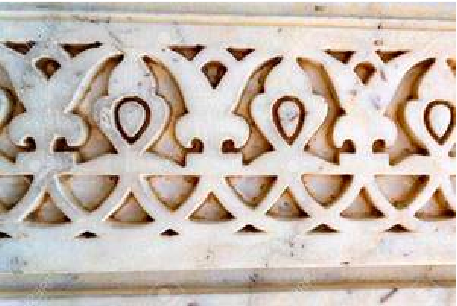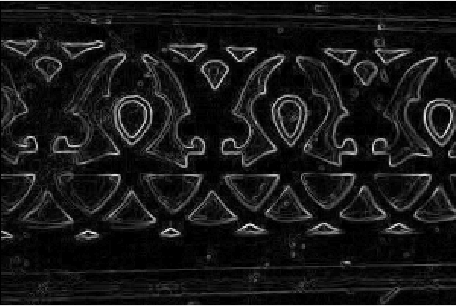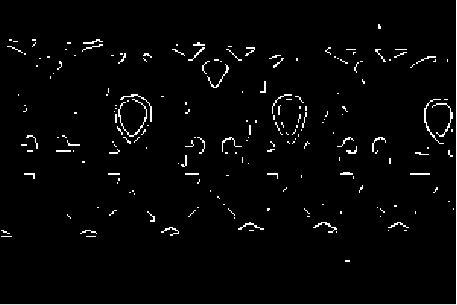# MATLAB – Image Edge Detection using Robert Operator from Scratch

• Last Updated : 17 May, 2020

Robert Operator: This gradient-based operator computes the sum of squares of the differences between diagonally adjacent pixels in an image through discrete differentiation. Then the gradient approximation is made. It uses the following 2 x 2 kernels or masks –Approach:

Step 1: Input – Read an image
Step 2: Convert the true-color RGB image to the grayscale image
Step 3: Convert the image to double
Step 4: Pre-allocate the filtered_image matrix with zeros
Step 5: Define Robert Operator Mask
Step 6: Edge Detection Process (Compute Gradient approximation and magnitude of vector)
Step 7: Display the filtered image
Step 8: Thresholding on the filtered image
Step 9: Display the edge-detected image

Implementation in MATLAB:

 % MATLAB Code | Robert Operator from Scratch  % Read Input Imageinput_image = imread('[name of input image file].[file format]');  % Displaying Input Imageinput_image = uint8(input_image);figure, imshow(input_image); title('Input Image');  % Convert the truecolor RGB image to the grayscale imageinput_image = rgb2gray(input_image);  % Convert the image to doubleinput_image = double(input_image);  % Pre-allocate the filtered_image matrix with zerosfiltered_image = zeros(size(input_image));  % Robert Operator MaskMx = [1 0; 0 -1];My = [0 1; -1 0];  % Edge Detection Process% When i = 1 and j = 1, then filtered_image pixel  % position will be filtered_image(1, 1)% The mask is of 2x2, so we need to traverse % to filtered_image(size(input_image, 1) - 1%, size(input_image, 2) - 1)for i = 1:size(input_image, 1) - 1    for j = 1:size(input_image, 2) - 1          % Gradient approximations        Gx = sum(sum(Mx.*input_image(i:i+1, j:j+1)));        Gy = sum(sum(My.*input_image(i:i+1, j:j+1)));                         % Calculate magnitude of vector        filtered_image(i, j) = sqrt(Gx.^2 + Gy.^2);             endend  % Displaying Filtered Imagefiltered_image = uint8(filtered_image);figure, imshow(filtered_image); title('Filtered Image');  % Define a threshold valuethresholdValue = 100; % varies between [0 255]output_image = max(filtered_image, thresholdValue);output_image(output_image == round(thresholdValue)) = 0;  % Displaying Output Imageoutput_image = im2bw(output_image);figure, imshow(output_image); title('Edge Detected Image');

Input Image –Filtered Image:Edge Detected Image: